Question
park confer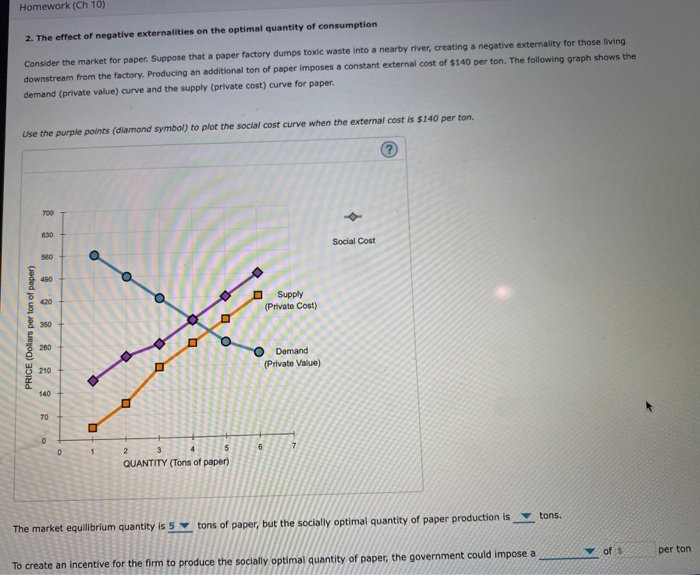To find the social cost, add \$140 to private cost at each level of output. Social cost=private cost+ marginal negative externality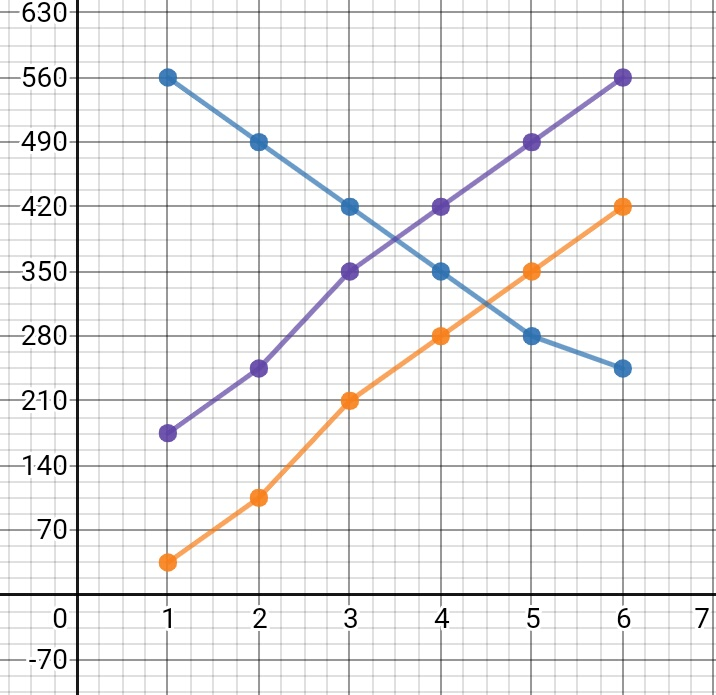Market equilibrium quantity is 4 tons of paper but socially optimal quantity of paper is 3.5 tons of paper.

Reason- Market equilibrium is where Demand =supply

Social equilibrium is where Social cost= Demand

The government could impose a TAX of \$140 per ton.

Reason- Tax will shift the supply curve upwards by \$140 producing the same effect as social supply curve.

If it helps kindly upvote

#### Earn Coins

Coins can be redeemed for fabulous gifts.

Similar Homework Help Questions
• ### Homework (Ch 10) 3. The effect of negative externalities on the optimal quantity of consumption Consider the market...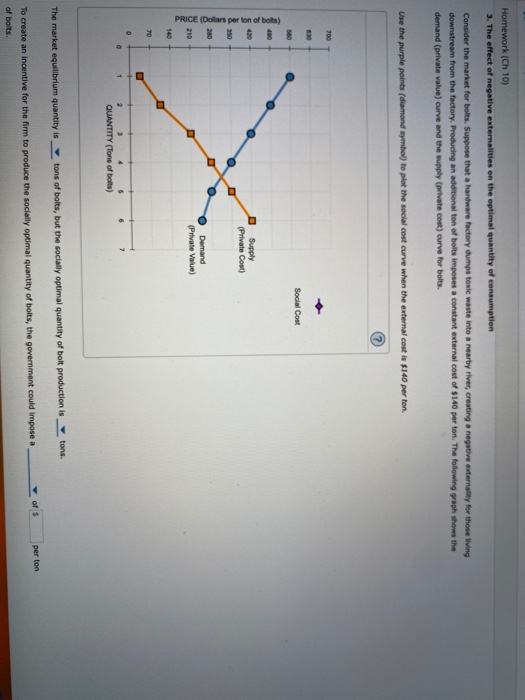Homework (Ch 10) 3. The effect of negative externalities on the optimal quantity of consumption Consider the market for bolts. Suppose that a hardware factory dumps toxic waste into a nearby river, creating a negative externality for those living downstream from the factory Producing an additional ton of bolts imposes a constant external cost of \$140 per ton. The following graph shows the demand (private value) curve and the supply (private cost) curve for bolts. Use the purple points (diamond...

• ### 3. The effect of negative externalities on the optimal quantity of consumption Consider the market for...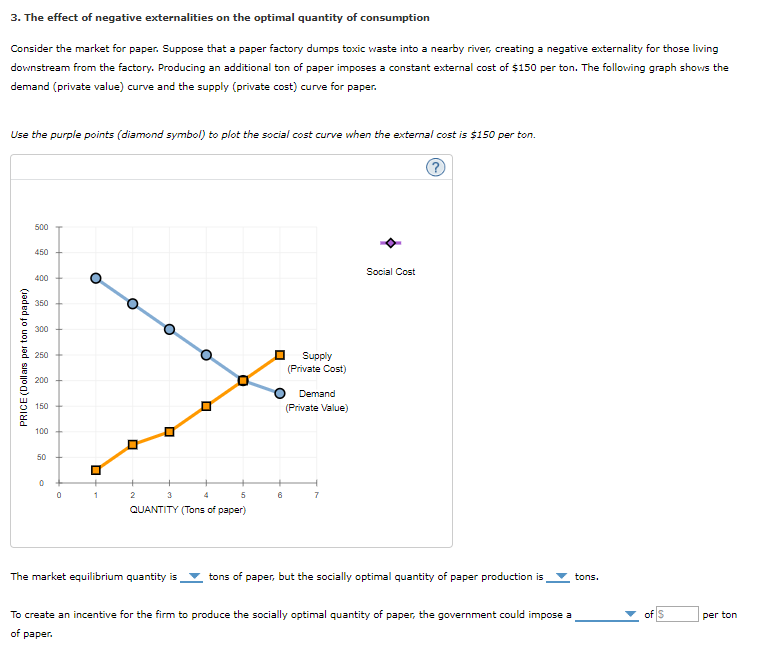3. The effect of negative externalities on the optimal quantity of consumption Consider the market for paper. Suppose that a paper factory dumps toxic waste into a nearby river, creating a negative externality for those living downstream from the factory. Producing an additional ton of paper imposes a constant external cost of \$150 per ton. The following graph shows the demand (private value) curve and the supply (private cost) curve for paper. Use the purple points (diamond symbol) to plot...

• ### 3. The effect of negative externalities on the optimal quantityof consumption Consider the market for paper....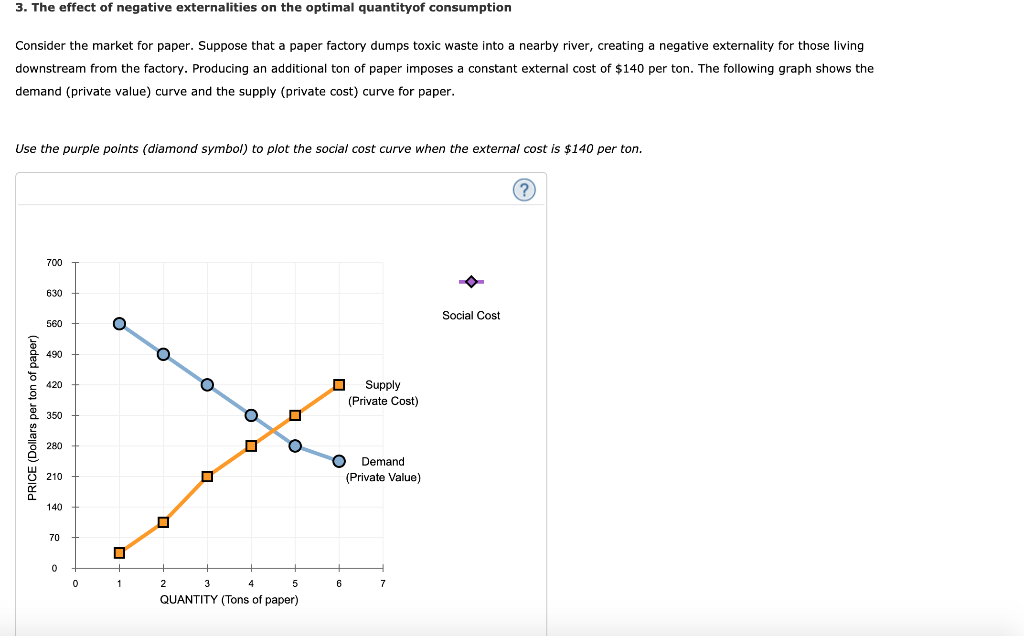3. The effect of negative externalities on the optimal quantityof consumption Consider the market for paper. Suppose that a paper factory dumps toxic waste into a nearby river, creating a negative externality for those living downstream from the factory. Producing an additional ton of paper imposes a constant external cost of \$140 per ton. The following graph shows the demand (private value) curve and the supply (private cost) curve for paper. Use the purple points (diamond symbol) to plot the...

• ### 3. The effect of negative externalities on the optimal quantity of consumption Consider the market for...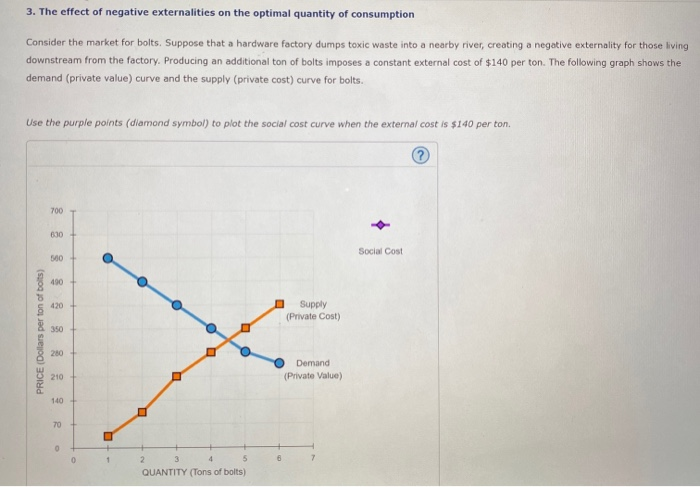3. The effect of negative externalities on the optimal quantity of consumption Consider the market for bolts. Suppose that a hardware factory dumps toxic waste into a nearby river, creating a negative externality for those living downstream from the factory. Producing an additional ton of bolts imposes a constant external cost of \$140 per ton. The following graph shows the demand (private value) curve and the supply (private cost) curve for bolts. Use the purple points (diamond symbol) to plot...

• ### Electric form is better. Thank you 3. The effect of negative externalities on the optimal quantity...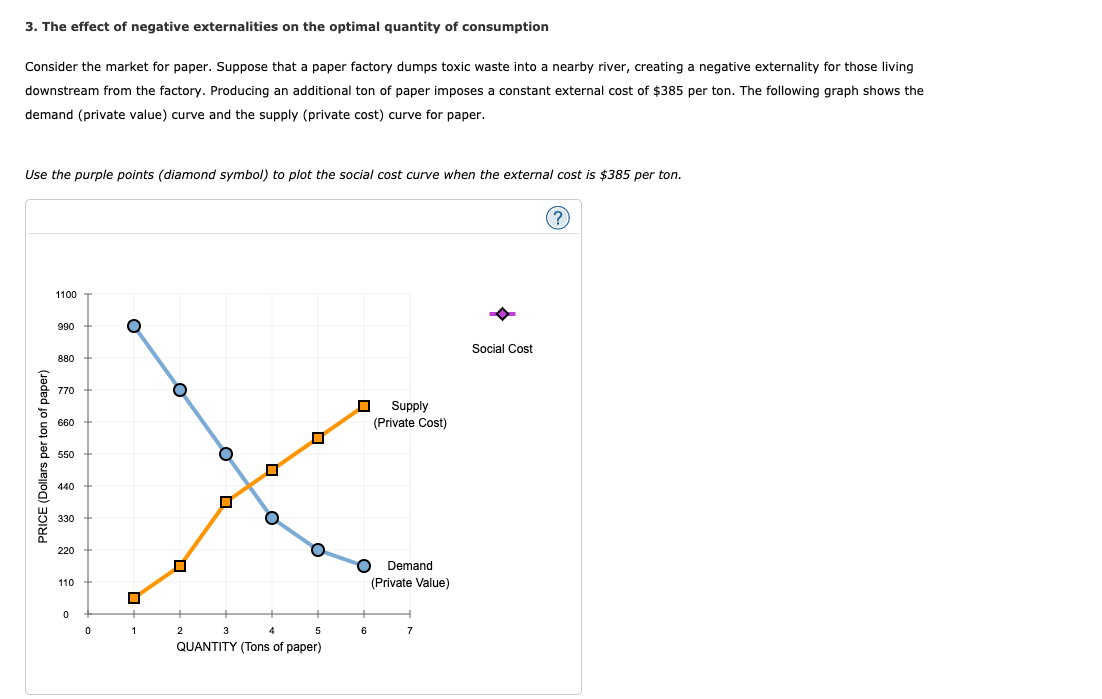Electric form is better. Thank you 3. The effect of negative externalities on the optimal quantity of consumption Consider the market for paper. Suppose that a paper factory dumps toxic waste into a nearby river, creating a negative externality for those living downstream from the factory. Producing an additional ton of paper imposes a constant external cost of \$385 per ton. The following graph shows the demand (private value) curve and the supply (private cost) curve for paper. Use the...

• ### Homework (Ch 10) 3. The effect of negative externalities on the optimal quantity of consumption Consider...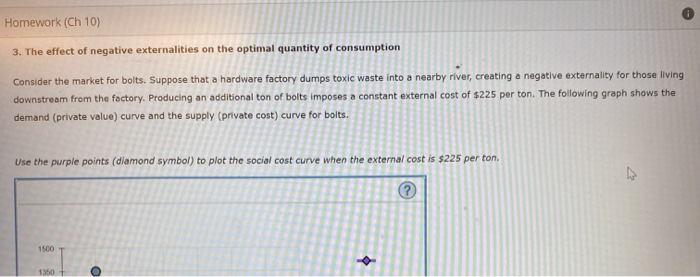Homework (Ch 10) 3. The effect of negative externalities on the optimal quantity of consumption Consider the market for bolts. Suppose that a hardware factory dumps toxic waste into a nearby river, creating a negative externality for those living downstream from the factory. Producing an additional ton of bolts imposes a constant external cost of \$225 per ton. The following graph shows the demand (private value) curve and the supply (private cost) curve for bolts. Use the purple points (diamond...

• ### 3. The effect of negative externalities on the optimal quantity of consumption Consider the market for...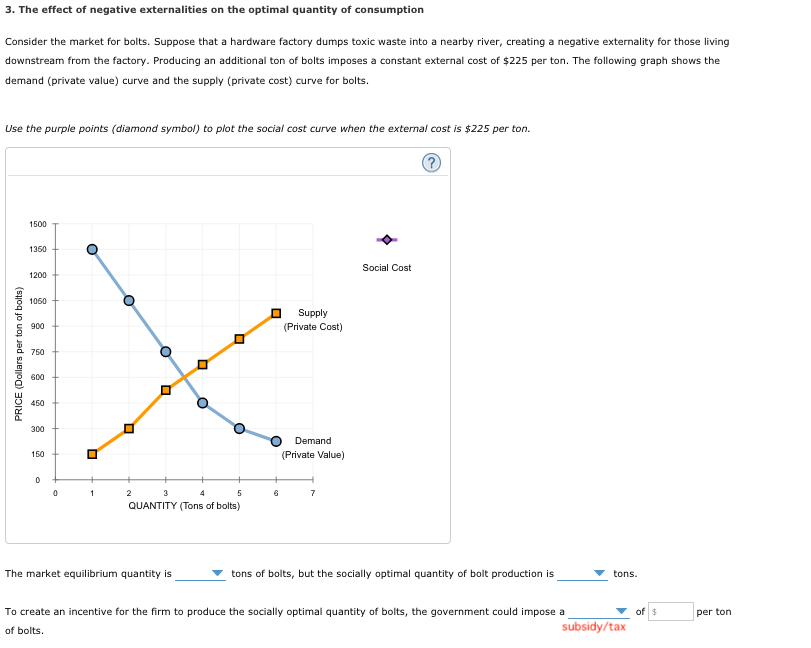3. The effect of negative externalities on the optimal quantity of consumption Consider the market for bolts. Suppose that a hardware factory dumps toxic waste into a nearby river, creating a negative externality for those living downstream from the factory. Producing an additional ton of bolts imposes a constant external cost of \$225 per ton. The following graph shows the demand (private value) curve and the supply (private cost) curve for bolts Use the purple points (diamond symbol) to plot...

• ### The effect of negative externalities on the optimal quantity of consumption Consider the market for bolts. Suppose that...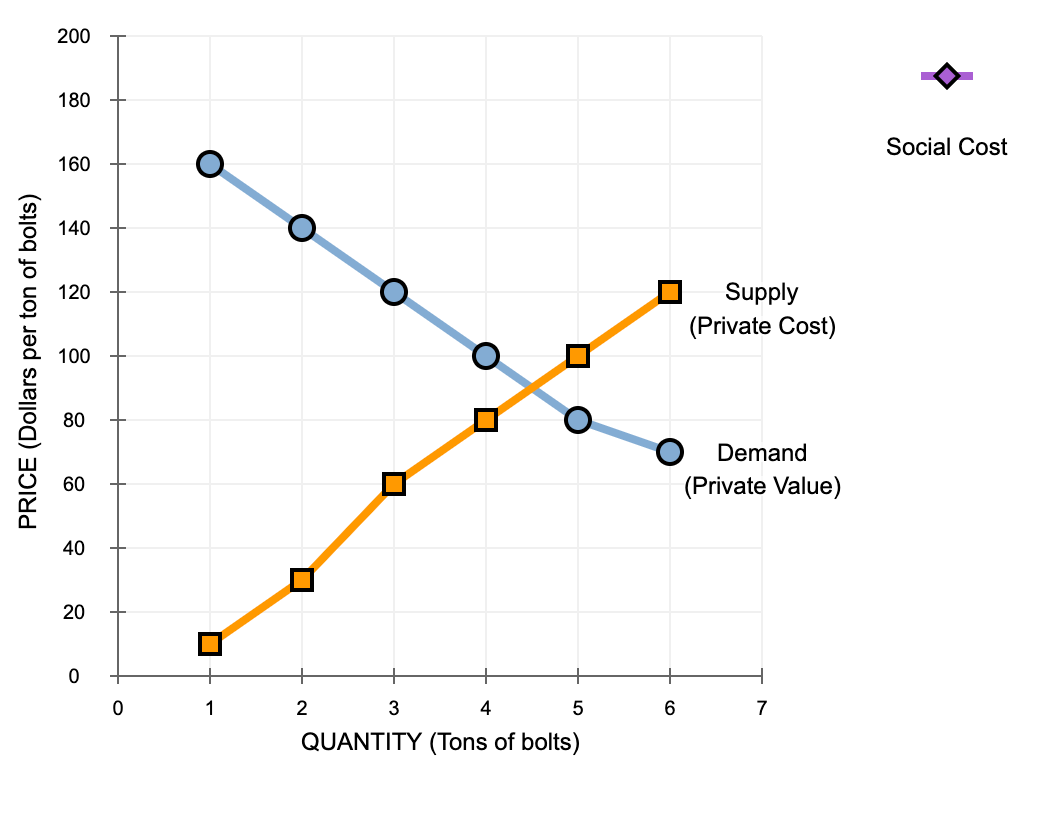The effect of negative externalities on the optimal quantity of consumption Consider the market for bolts. Suppose that a hardware factory dumps toxic waste into a nearby river, creating a negative externality for those living downstream from the factory. Producing an additional ton of bolts imposes a constant external cost of \$40 per ton. The following graph shows the demand (private value) curve and the supply (private cost) curve for bolts. 1. plot the social cost curve when the external...

• ### The effect of negative externalities on the optimal quantityof consumption Consider the market for paper. Suppose that...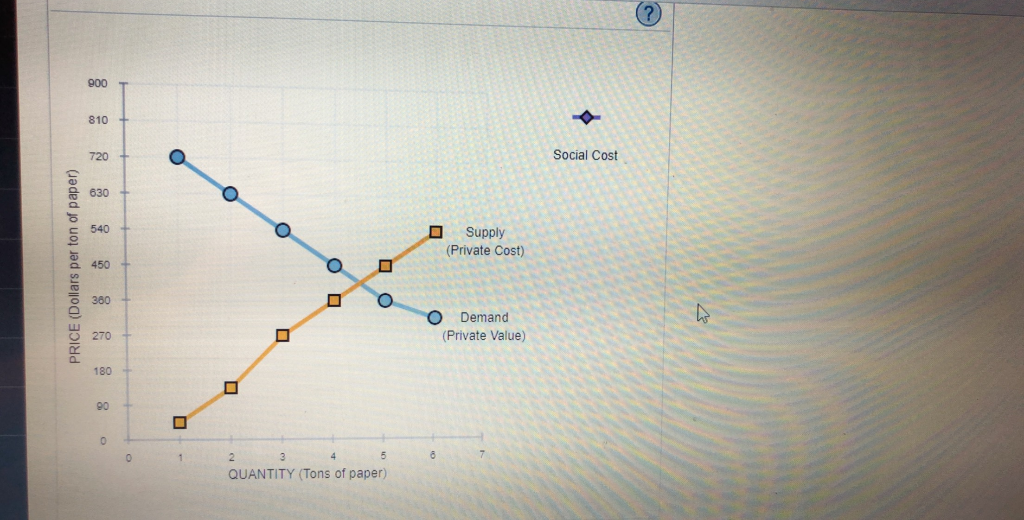The effect of negative externalities on the optimal quantityof consumptionConsider the market for paper. Suppose that a paper factory dumps toxic waste into a nearby river, creating a negative externality for those living downstream from the factory. Producing an additional ton of paper imposes a constant external cost of \$180 per ton. The following graph shows the demand (private value) curve and the supply (private cost) curve for paper.Use the purple points (diamond symbol) to plot the social cost curve...

• ### 3. The effect of negative externalities on the optimal quantity of consumption Consider the market for...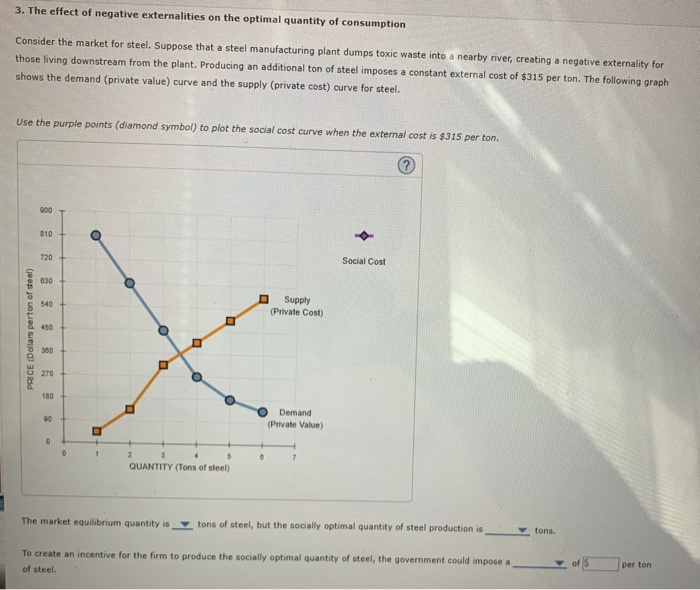3. The effect of negative externalities on the optimal quantity of consumption Consider the market for steel. Suppose that a steel manufacturing plant dumps toxic waste into a nearby river, creating a negative externality for those living downstream from the plant. Producing an additional ton of steel imposes a constant external cost of \$315 per ton. The following graph shows the demand (private value) curve and the supply (private cost) curve for steel. Use the purple points (diamond symbol) to...